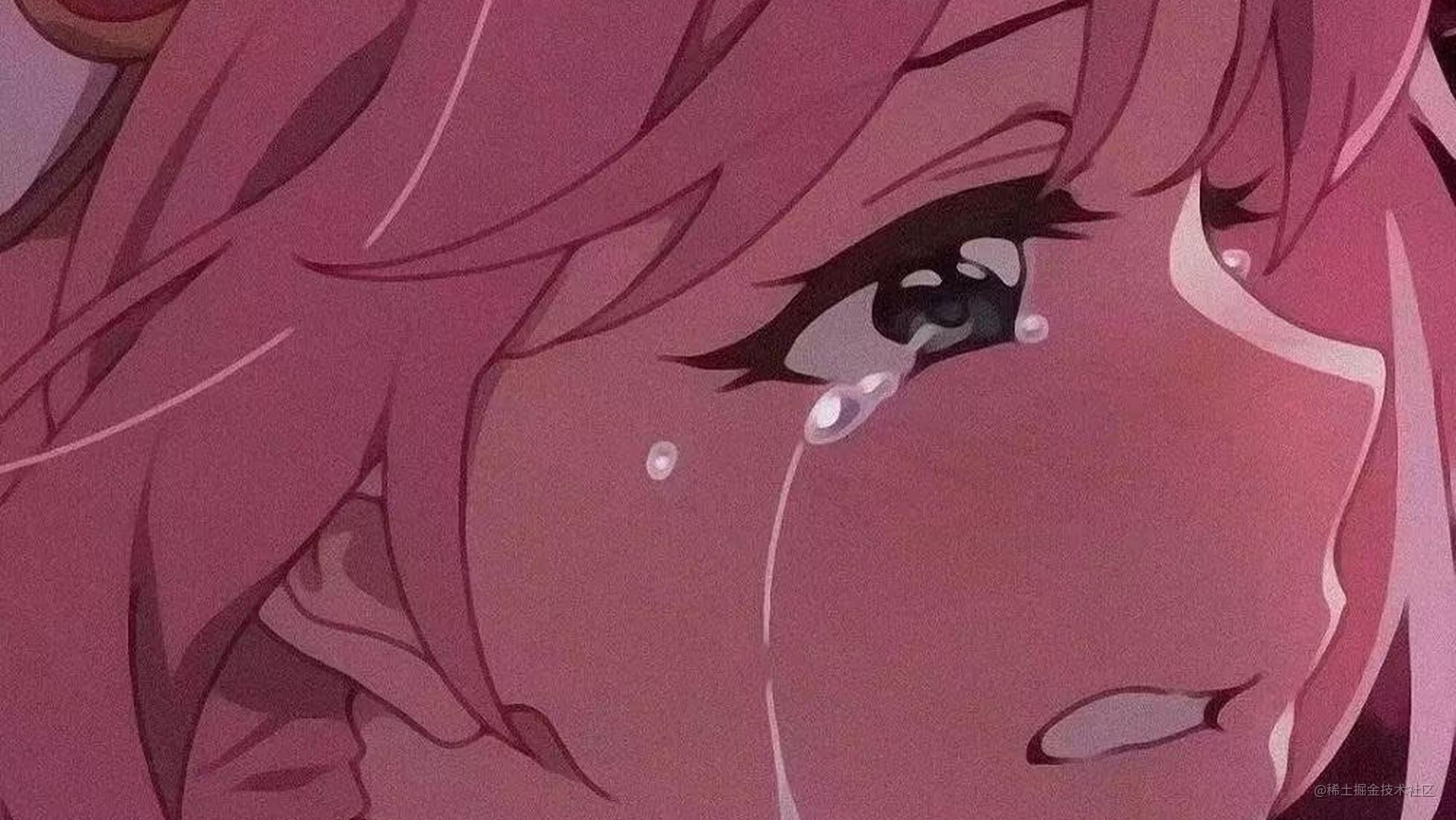# 原生JS图片拖动、缩放、边界等问题总结

## 前言

### 1. 图片拖动

``````<div class="box">
<div class="avatar">
<img src="./avatar1.jpg" draggable="false">
</div>
</div>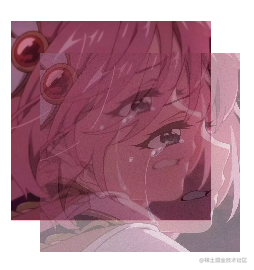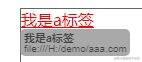`document.addEventListener('selectstart', e => { e.preventDefault() })`

##### 鼠标按下

``````const mouseDown = e => {
let transf = getTransform(oDiv)
x = e.clientX - transf.transX // 图片初始位置
y = e.clientY - transf.transY // 图片初始位置
}

##### 鼠标拖动

``````const mouseMove = e => {
let multiple = getTransform(oDiv).multiple
let moveX = e.clientX - x // x向移动距离
let moveY = e.clientY - y // y向移动距离
let newTransf = limitBorder(oDiv, oBox, moveX, moveY, multiple)
oDiv.style.transform = `matrix(\${multiple}, 0, 0, \${multiple}, \${newTransf.transX}, \${newTransf.transY})`
}

##### 鼠标抬起

``````const mouseUp = () => {
document.removeEventListener('mousemove', mouseMove)
document.removeEventListener('mouseup', mouseUp)
}

##### 鼠标滚轮滚动

``````const zoom = e => {
let transf = getTransform(oDiv)
if (e.deltaY < 0) {
transf.multiple *= DELTA // 放大DELTA倍
} else {
transf.multiple /= DELTA // 缩小DELTA倍
}
let newTransf = limitBorder(oDiv, oBox, transf.transX, transf.transY, transf.multiple)
oDiv.style.transform = `matrix(\${transf.multiple}, 0, 0, \${transf.multiple}, \${newTransf.transX}, \${newTransf.transY})`
}

### 2. 边界问题

`transX = Math.max(Math.min(moveX, right), left)`

`transY = Math.max(Math.min(moveY, bottom), top)`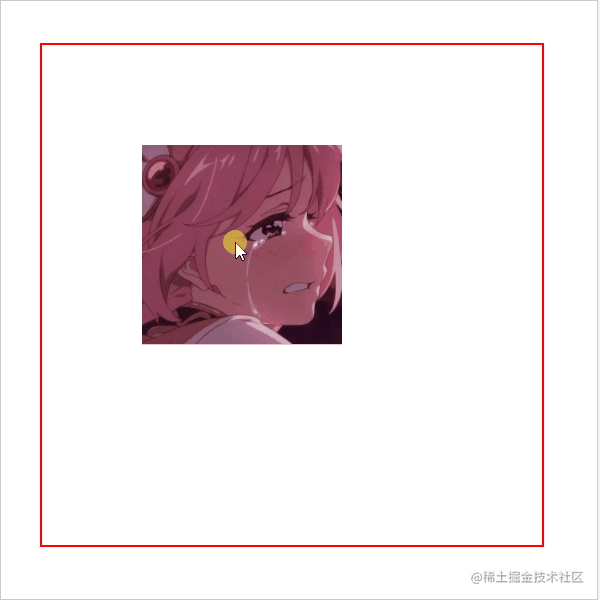``````const limitBorder = (innerDOM, outerDOM, moveX, moveY, multiple) => {
let { clientWidth: innerWidth, clientHeight: innerHeight, offsetLeft: innerLeft, offsetTop: innerTop } = innerDOM
let { clientWidth: outerWidth, clientHeight: outerHeight } = outerDOM
let transX
let transY
// 放大的图片超出box时 图片最多拖动到与边框对齐
if (innerWidth * multiple > outerWidth || innerHeight * multiple > outerHeight) {
if (innerWidth * multiple > outerWidth && innerWidth * multiple > outerHeight) {
transX = Math.min(Math.max(moveX, outerWidth - innerWidth * (multiple + 1) / 2 - innerLeft), -innerLeft + innerWidth * (multiple - 1) / 2)
transY = Math.min(Math.max(moveY, outerHeight - innerHeight * (multiple + 1) / 2 - innerTop), -innerTop + innerHeight * (multiple - 1) / 2)
} else if (innerWidth * multiple > outerWidth && !(innerWidth * multiple > outerHeight)) {
transX = Math.min(Math.max(moveX, outerWidth - innerWidth * (multiple + 1) / 2 - innerLeft), -innerLeft + innerWidth * (multiple - 1) / 2)
transY = Math.max(Math.min(moveY, outerHeight - innerHeight * (multiple + 1) / 2 - innerTop), -innerTop + innerHeight * (multiple - 1) / 2)
} else if (!(innerWidth * multiple > outerWidth) && innerWidth * multiple > outerHeight) {
transX = Math.max(Math.min(moveX, outerWidth - innerWidth * (multiple + 1) / 2 - innerLeft), -innerLeft + innerWidth * (multiple - 1) / 2)
transY = Math.min(Math.max(moveY, outerHeight - innerHeight * (multiple + 1) / 2 - innerTop), -innerTop + innerHeight * (multiple - 1) / 2)
}
}
// 图片小于box大小时 图片不能拖出边框
else {
transX = Math.max(Math.min(moveX, outerWidth - innerWidth * (multiple + 1) / 2 - innerLeft), -innerLeft + innerWidth * (multiple - 1) / 2)
transY = Math.max(Math.min(moveY, outerHeight - innerHeight * (multiple + 1) / 2 - innerTop), -innerTop + innerHeight * (multiple - 1) / 2)
}
return { transX, transY }
}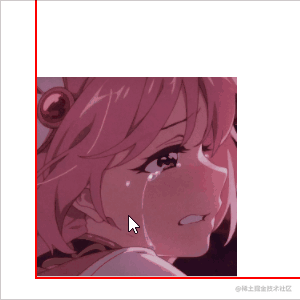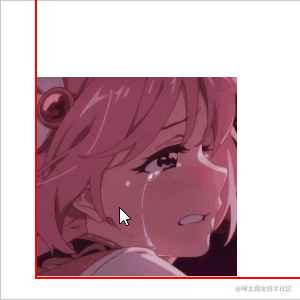### 3. 获取transform

##### `getComputedStyle` 以及 `matrix` 矩阵

Window.getComputedStyle()方法返回一个对象，该对象在应用活动样式表并解析这些值可能包含的任何基本计算后报告元素的所有CSS属性的值。 私有的CSS属性值可以通过对象提供的API或通过简单地使用CSS属性名称进行索引来访问。

MDN官方的解释比较难懂，其实简单来说 `getComputedStyle(DOM).transform` 会得到一个矩阵 `matrix(1,0,0,1,100,100)`，在没有旋转`rotate`、拉伸`skew`的情况下，我们只需要关注括号里的1、4、5、6位，分别代表`x`向缩放倍数、`y`向缩放倍数、`x`向偏移量和`y`向偏移量。这种统一形式的字符串，我们完全可以用`split`分隔为数组再依次取到

``````const getTransform = DOM => {
let arr = getComputedStyle(DOM).transform.split(',')
return {
transX: isNaN(+arr[arr.length - 2]) ? 0 : +arr[arr.length - 2], // 获取translateX
transY: isNaN(+arr[arr.length - 1].split(')')) ? 0 : +arr[arr.length - 1].split(')'), // 获取translateX
multiple: +arr // 获取图片缩放比例
}
}